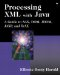# Fibonacci Numbers

### Fibonacci Numbers

As far as we know, the Fibonacci series was first discovered by Leonardo of Pisa about 1200 C.E. Leonardo was trying to answer the question, " Quot paria coniculorum in uno anno ex uno pario germinatur? " or, in English, "How many pairs of rabbits are born in one year from one pair?" To solve his problem, Leonardo estimated that rabbits have a one-month gestation period and can first mate at the age of one month, so that each female rabbit has its first litter at two months. He made the simplifying assumption that each litter consisted of exactly one male and one female .

Leonardo's analysis begins with one pair of baby rabbits, a male and a female. At the end of the first month, these two have reached puberty and mate. There is still one pair of rabbits. At the end of the second month, the female gives birth to a new pair of rabbits. There are now two pairs of rabbits, one pair of adults and one pair of babies. The adult pair mates again, so that they produce one more pair at the end of the third month, at which point there are three pairs of rabbits. One of these pairs has just been born, but the other pairs are old enough to mate, which, being rabbits, they do. At the end of the fourth month, two of the three pairs have babies, so that there are now five pairs of rabbits. Meanwhile, all rabbits born by the previous month mate, so that at the end of the fifth month there are three more pairs of rabbits.

Leonardo realized that the number of pairs at the end of each month equaled the sum of the number of pairs the preceding month and the number of pairs the month before that. The rabbits don't simply double in population each month, because it takes two months before a rabbit can have its first litter. Nonetheless, the numbers do grow only slightly more slowly than exponentially; and the process continues indefinitely, at least until you run out of rabbit food or the rabbits take over the world, whichever comes first. The sequence representing the number of pairs each month1, 1, 2, 3, 5, 8, 13, has become known as the Fibonacci series after Leonardo's Latin nickname, Fibonacci (short for filius Bonacci, son of Bonacci).

The rabbits aren't so important, but the math is. Each integer in the series is formed by the sum of the two previous integers. The first two integers in the series are 1 and 1.  The Fibonacci series turns up in some very unexpected places, including decimal expansions of p , the golden ratio, the Mandelbrot set, the number of petals on many flowers, the number of spirals on pine cones and pineapples, the size of chambers in a nautilus shell, and many more.

 In some variations, the first two integers are 0 and 1. Aside from the initial zero, this produces the same series.

It's very easy to calculate the Fibonacci sequence by computer. A simple for loop will do. For example, this code fragment prints the first 40 Fibonacci numbers:

` int low = 1;  int high = 1; for (int i = 1; i < 40; i++) {   System.out.println(low);   int temp = high;   high = high+low;   low = temp; } `

However, the Fibonacci numbers do grow very large very quickly, and exceed the bounds of an int shortly before the fiftieth generation. Consequently, it's better to use the java.math.BigInteger class instead, as Example 3.1 demonstrates .

##### Example 3.1 A Program That Calculates the Fibonacci Numbers
` import java.math.BigInteger; public class FibonacciNumbers {   public static void main(String[] args) {     BigInteger low  = BigInteger.ONE;     BigInteger high = BigInteger.ONE;     for (int i = 1; i <= 10; i++) {       System.out.println(low);       BigInteger temp = high;       high = high.add(low);       low = temp;     }   } } `

When run, Example 3.1 produces the first ten Fibonacci numbers:

` C:\XMLJAVA>  java FibonacciNumbers  1 1 2 3 5 8 13 21 34 55 `

It would be straightforward to read the number of Fibonacci numbers to generate from the command line. But because user interfaces aren't the focus of this book, I don't want to obscure the important parts with too much extraneous fluff.Processing XML with Javaв„ў: A Guide to SAX, DOM, JDOM, JAXP, and TrAX
ISBN: 0201771861
EAN: 2147483647
Year: 2001
Pages: 191